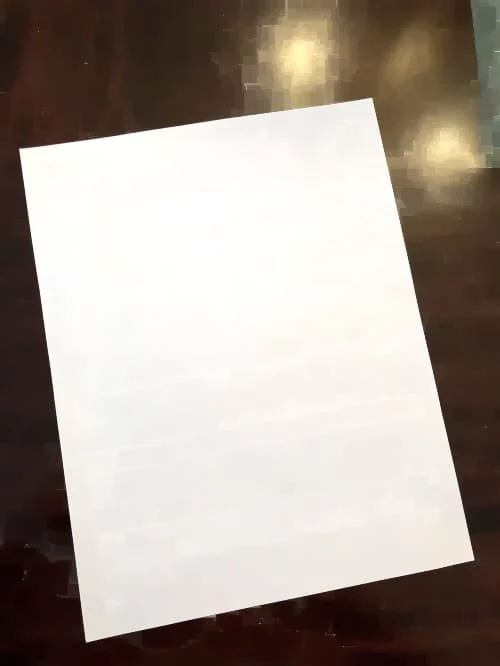﻿ 一文学会使用OpenCV构建文档扫描仪_python_脚本之家
python# 一文学会使用OpenCV构建文档扫描仪

## 介绍

OpenCV 的简要概述： OpenCV 是一个开源库，用于各种计算机语言的图像处理，包括 Python、C++ 等。它可用于检测照片（例如使用人脸检测系统的人脸） 。• 形态学运算

• 边缘和轮廓检测

• 识别角点

• 转变视角

## 执行形态学操作

```kernel = np.ones((5,5),np.uint8)
img = cv2.morphologyEx(img, cv2.MORPH_CLOSE, kernel, iterations= 3)```## 从捕获的图像中删除背景

GrabCut 在接收到输入图片及其边界后，剔除边界外的所有元素。

```mask = np.zeros(img.shape[:2],np.uint8)
bgdModel = np.zeros((1,65),np.float64)
fgdModel = np.zeros((1,65),np.float64)
rect = (20,20,img.shape-20,img.shape-20)## 边缘和轮廓检测

（注意：Canny 函数仅适用于灰度图像，因此如果图像尚不存在，则将图像转换为灰度）。

```gray = cv2.cvtColor(img, cv2.COLOR_BGR2GRAY)
gray = cv2.GaussianBlur(gray, (11, 11), 0)
# Edge Detection.
canny = cv2.Canny(gray, 0, 200)
canny = cv2.dilate(canny, cv2.getStructuringElement(cv2.MORPH_ELLIPSE, (5, 5)))```

```gray = cv2.cvtColor(img, cv2.COLOR_BGR2GRAY)
gray = cv2.GaussianBlur(gray, (11, 11), 0)
# Edge Detection.
canny = cv2.Canny(gray, 0, 200)
canny = cv2.dilate(canny, cv2.getStructuringElement(cv2.MORPH_ELLIPSE, (5, 5)))```## 识别角点

```con = np.zeros_like(img)
# Loop over the contours.
for c in page:
# Approximate the contour.
epsilon = 0.02 * cv2.arcLength(c, True)
corners = cv2.approxPolyDP(c, epsilon, True)
# If our approximated contour has four points
if len(corners) == 4:
break
cv2.drawContours(con, c, -1, (0, 255, 255), 3)
cv2.drawContours(con, corners, -1, (0, 255, 0), 10)
corners = sorted(np.concatenate(corners).tolist())
for index, c in enumerate(corners):
character = chr(65 + index)
cv2.putText(con, character, tuple(c), cv2.FONT_HERSHEY_SIMPLEX, 1, (255, 0, 0), 1, cv2.LINE_AA)```## 标准化四点定位

```def order_points(pts):
rect = np.zeros((4, 2), dtype='float32')
pts = np.array(pts)
s = pts.sum(axis=1)
rect = pts[np.argmin(s)]
rect = pts[np.argmax(s)]
diff = np.diff(pts, axis=1)
rect = pts[np.argmin(diff)]
rect = pts[np.argmax(diff)]
return rect.astype('int').tolist()```

## 寻找目的地坐标：

```(tl, tr, br, bl) = pts
# Finding the maximum width.
widthA = np.sqrt(((br - bl) ** 2) + ((br - bl) ** 2))
widthB = np.sqrt(((tr - tl) ** 2) + ((tr - tl) ** 2))
maxWidth = max(int(widthA), int(widthB))
# Finding the maximum height.
heightA = np.sqrt(((tr - br) ** 2) + ((tr - br) ** 2))
heightB = np.sqrt(((tl - bl) ** 2) + ((tl - bl) ** 2))
maxHeight = max(int(heightA), int(heightB))
# Final destination co-ordinates.
destination_corners = [[0, 0], [maxWidth, 0], [maxWidth, maxHeight], [0, maxHeight]]```

## 透视变换

```# Getting the homography.
M = cv2.getPerspectiveTransform(np.float32(corners), np.float32(destination_corners))
final = cv2.warpPerspective(orig_img, M, (destination_corners, destination_corners), flags=cv2.INTER_LINEAR)```